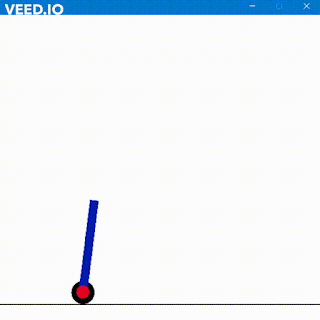### Verilog Code for 8bit Full Adder

Hola Amigos
Adders as we all have used might pose a problem when simulated for very long space complex projects as the results of full adder are not instant. It does take time and the propagation delay increases with time so how do we deal with delay caused by each gate. Here have a look on this basic adder

What we come to know about it is that for C4 we have to wait for C3, similarly for C3 we have to wait for C2. This causes delay and then there comes Carry Look Ahead Adder in limelight.

One can increase the space complexity of the code to decrease the time for propagation or introduce just faster delays to decrease the propagation delay of the overall adder.

A basic full adder is a parallel adder which is based on complex circuit to directly induce the carry from the inputs provided.

Now S = Sum and C(-1) = carry from previous addition.
So S = EXOR(A,B,C) and
C(0) = A.B + EXOR(A,B).C(-1)

Now let  A.B = Gi  and EXOR(A,B) = Pi

So        C0  = G0 + P0 . C-1

C1  = G1 + Pi . C0

C2  = G2 + Pi . C1

C3  = G3 + Pi . C2

C4  = G4 + Pi . C2

#### Code for a 8 bit Full Adder

module FA(a,b,c,s,co);
input [7:0]a,b;
output [8:0]s;
output co;
input c;
Carry c1(a,b,c,s,cx0);
Carry c2(a,b,cx0,s,cx1);
Carry c3(a,b,cx1,s,cx2);
Carry c4(a,b,cx2,s,cx3);
Carry c5(a,b,cx3,s,cx4);
Carry c6(a,b,cx4,s,cx5);
Carry c7(a,b,cx5,s,cx6);
Carry c8(a,b,cx6,s,s);
assign co = s;
endmodule

module Carry(a,b,c,s,co);
input a,b;
input c;
output s,co;
wire out4;
wire out6;
and (out1,a,b);
xor (out2,a,b);
and (out3,out2,c);
or (out4,out3,out1);
assign co = out4;
xor (out5,a,b);
xor (out6,out5,c);
assign s = out6;
endmodule

module TestCarry;

// Inputs
reg [7:0] a;
reg [7:0] b;
reg c;
wire [8:0] s;
wire co;
CLA uut (
.a(a),
.b(b),
.c(c),
.s(s),
.co(co)
);
initial begin
// Initialize Inputs
a = 8'b01101010;
b = 8'b10111101;
c = 0;
end
endmodule

Here is the output in action

Here s[8:0] is the sum c is input carry co is the final carry a[7:0] and b[7:0] are the numbers

So Long

1.I get the following error when running :

testbench.sv:11: error: Unknown module type: CLA
2 error(s) during elaboration.
*** These modules were missing:
CLA referenced 1 times.
***
Exit code expected: 0, received: 1

1.Replace CLA with FA in testbench and try.
Let me know the result

### Self Balancing Robot using Machine Learning

Hola Amigos, I have always loved inverted pendulums. They are very fascinating to me and I play with them a lot. In real life, I have m...